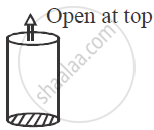Advertisement Remove all ads

# Show that the Height of a Cylinder, Which is Open at the Top, Having a Given Surface Area and Greatest Volume, is Equal to the Radius of Its Base. - Mathematics

Sum

Show that the height of a cylinder, which is open at the top, having a given surface area and greatest volume, is equal to the radius of its base.

Advertisement Remove all ads

#### Solution

Given a cylinder

S = 2πrh + πr            .......(1)

V = πrh                    .......(2 )From (1) S - πr2 = 2 πrh

h = [(S -pir^2)/(2pir)]  = S/(2pir) - r/2

Put in (2)

V =  pir^2h = pir^2 [ S/(2pir) - r/2] = (Sr)/2 - (pir^3)/2

Now diff on both sides by ‘r’

(dv)/(dr) = S/2 - (3pir^2)/2

For max/min (dv)/(dr) = 0

S/2 - (3pir^2)/2 = 0 ⇒ S = 3pir^2      ........(3)

(d^2 v)/(dr^2) = -3pir = -3 pi x sqrt(S/3pi) <0

∴ By second derivative test it is maxima from (1) & (3)

2pirh + pir^2 = 3pir^2

2pir h = 2 pir^2

h = r

Is there an error in this question or solution?
Advertisement Remove all ads

#### APPEARS IN

Advertisement Remove all ads

#### Video TutorialsVIEW ALL 

Advertisement Remove all ads
Share
Notifications

View all notifications

Forgot password?